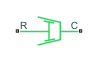# Nonlinear Rotational Damper

Rotational damper based on polynomial or table lookup parameterizations

## Library

Simscape / Driveline / Couplings & Drives / Springs & Dampers

•## Description

The block represents a nonlinear rotational damper. Polynomial and table lookup parameterizations define the nonlinear relationship between damping torque and relative angular velocity. The damping torque can be symmetric or asymmetric about the zero velocity point. The block applies equal and opposite damping torques on the two rotational conserving ports.

The symmetric polynomial parameterization defines the damping torque for both positive and negative relative velocities according to the expression:

`$T={b}_{1}\omega +sign\left(\omega \right)\cdot {b}_{2}{\omega }^{2}+{b}_{3}{\omega }^{3}+sign\left(\omega \right)\cdot {b}_{4}{\omega }^{4}+{b}_{5}{\omega }^{5},$`

where:

• T — Damping torque

• b1, b2, ..., b5 — Damping coefficients

• ω — Relative angular velocity between ports R and C, $\omega ={\omega }_{R}-{\omega }_{C}$

• ωR — Absolute angular velocity associated with port R

• ωC — Absolute angular velocity associated with port C

To avoid zero-crossings that slow simulation, eliminate the sign function from the polynomial expression by specifying an odd polynomial (b2,b4 = 0).

The two-sided polynomial parameterization defines the damping torque for both positive and negative relative velocities according to the expression:

`$T=\left\{\begin{array}{cc}{b}_{1p}\omega +{b}_{2p}{\omega }^{2}+{b}_{3p}{\omega }^{3}+{b}_{4p}{\omega }^{4}+{b}_{5p}{\omega }^{5},& \omega \ge 0\\ {b}_{1n}\omega +{b}_{2n}{\omega }^{2}+{b}_{3n}{\omega }^{3}+{b}_{4n}{\omega }^{4}+{b}_{5n}{\omega }^{5},& \omega <0\end{array},$`

where:

• b1p, b2p, ..., b5p — Damping coefficients for positive relative velocities

• b1n, b2n, ..., b5n — Damping coefficients for negative relative velocities

Both polynomial parameterizations use a fifth-order polynomial expression. To use a lower-order polynomial, set the unneeded higher-order coefficients to zero. To use a higher-order polynomial, fit to a lower-order polynomial or use the table lookup parameterization.

The table lookup parameterization defines damping torque based on a set of torque and angular velocity vectors. If not specified, the block automatically adds a data point at the origin (zero angular velocity and zero torque).

### Assumptions and Limitations

The block assumes viscous damping. The damping force depends only on velocity.

## Ports

`C`

Rotational conserving port.

`R`

Rotational conserving port.

## Parameters

Parameterization

Select damping parameterization. Options are ```By polynomial``` and ```By table lookup```.

## Version History

Introduced in R2013a